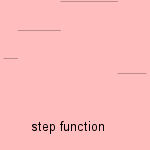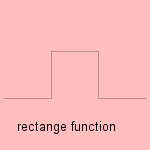# step function

## line

last updated: 2005-11-13Using only horizontal line segments 1), the step or staircase function is formed. Every discrete function can be seen as such a step function.

Another function that is sometimes called the step function, is the entier function int(x) or [x]. Now the steps are of equal length: the function gives for each x the first lower natural number. The entier function can be used to form the triangle curve.

For esthetic purposes the horizontal line segments can be connect by vertical segments.
This conflicts with on the properties of a function, being that for each value of x there is not more than one function value.The function of Heaviside Ha() has only one step: until x = a it has a value zero, above the value one. The function describes the velocity of an object as result of a sudden force, during a infinitely small time interval. H is the abbreviated notation for H0, what is in fact the same as the unit step function. At the place of the step the value of the function can be set to ˝.
This is in fact the same function as the sign function or signum function sgn(x) = |x| / x, which gives the sign of a number.The rectangle function P(x) is equal to 0 outside an interval, and equal to 1 inside. It can be expressed as the difference of two functions of Heaviside.
The curve is also called pulse function, gate function, or window function.
The boxcar function Bc(a,b) is a variant on this theme: value c for a <= x <= b and 0 otherwise.

The Fourier transform of the function is the sinc function.

When taking only integer values for x, we get the discrete curves.

notes

1) By the way, because it's the case of a function, vertical line segments are not admitted.